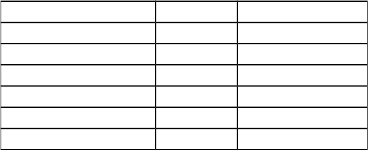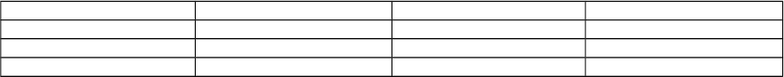# W08 Final 3352.doc

71 views10 pagesQuestion 1 (15 points)
You have \$10,000 to invest for one period (one year). You can invest in a Treasury bill to earn 4% per year. You can
also invest in an ETF (exchange traded fund) that traces the S&P 500 index. You can buy any fraction of the ETF.
Assume that the ETF will have with two possible outcomes at the end of the year: it will be worth \$150 if the state of
economy is good; it will be worth \$100 if the state of economy is bad. Dividends on the ETF can be ignored. The
probabilities of the good and bad states are 0.3 and 0.7, respectively.
(1) (2 points) What is the expected value of the ETF at the year end?
(2) (2 points) What is the standard deviation of the ETF?
(3) (3 points) What is the fair market value of the ETF if it implies 11% risk premium per year?
(4) (3 points) If you require 9% expected return on your complete portfolio, how should you allocate between the
risk-free and the risky assets?
(5) (3 points) If you require an expected return of 20% per year, and you are allowed to borrow money at the 5%
interest rate, how can you achieve it (be numerically specific)?
(6) (2 points) Following (5) but assuming that you can only borrow money at 6% interest rate, how can you achieve
it? Explain whether the standard deviation of your complete portfolio is higher or lower than that in (5).
(Extra space on next page)
Question 2 (15 points)
Consider the information about two risky assets and one risk-free asset:
%12)(
1
=
rE
,
%18)(
2
=
rE
,
%15
1
=
σ
,
%20
2
=
σ
,
2
21
(%)60),cov(
=
rr
(i.e., 0.006),
%5=
f
r
(0.05)
You are encouraged to draw relevant graphs to help solving the problem.
(1) ( 5 points) Identify the minimum variance portfolio and find the expected return and standard deviation.
(2) (5 points) Identify the optimal portfolio and find the expected return and standard deviation.
(3) (3 points) An investor has allocated all of his wealth in risky assets 1 and 2 with 75% and 25% , respectively.
How should you advice the investor to maximize his expected return without increasing the standard deviation of
his portfolio? Be numerically specific.
(4) (2 points) Following (3), how should you advice the investor to minimize his standard deviation of expected
return without decreasing his return? Be numerically specific.
Question Credit Mark
115
215
315
415
Multiple Choice 40
Total 100
Unlock document

This preview shows pages 1-3 of the document.
Unlock all 10 pages and 3 million more documents.

Already have an account? Log inQuestion 3 (15 points)
The risk-free interest rate is 5%, and the expected return of a passive equity portfolio is 12% with standard deviation
of 20%. You wish to establish an active sub-portfolio then combine it with the passive portfolio to form an optimal
portfolio. You have a total of \$50,000 to invest. You want invest 30% in risk-free asset, and the rest in the optimal
portfolio.
You are examining the following three individual stocks:
Stock Expected Return Beta Residual std. dev.
A 0.250 2.5 0.7
B 0.200 1.6 0.6
C 0.150 1.2 0.5
(1) (5 points) Calculate the weights for the active portfolio.
(2) (5 points) Calculate the alpha, beta, and residual standard deviation of the active portfolio.
(3) (5 points) Determine the exact allocation in dollar term for every asset in the complete portfolio.
(Extra space on the next page)
Question 4 (15 points)
Company XYZ has recently announced annual earnings of \$2.00 per share, and paid an annual dividend of \$0.50 per
share. This company has a beta of 1.3. The riskfree interest rate is 5% per year, and the market risk premium is 7%
per year. This company has had a steady dividend growth of 10% per year in the past. This company has also
experienced pleasant earnings growth in the last 5 years at 20% per year.
(a) (5 points) If the dividend growth trend continues indefinitely, what is the intrinsic value per share of Company
XYZ?
(b) (10 points) The management of Company XYZ has communicated with shareholders that the earnings growth of
20% per year in the last 5 years is largely attributable to the small dividend payout ratio. The management predicts
that the 20% earnings growth will continue for the next 5 years while maintaining the current level of dividend
growth. Starting at year 6, the company can afford to increase the dividend payoff ratio to 50% and maintain the
dividend growth at 10% per year thereafter. Based on this communication, what is the intrinsic value per share of
Company XYZ?
Part II: 40 Multiple Choice Questions, 1 point each
1. You sold ABC stock short at \$80 per share. Your losses could be minimized by placing a __________:
A) limit-sell order
B) limit-buy order
C) stop-buy order
D) day-order
E) none of the above.
2. Shares for short transactions
A) are usually borrowed from other brokers.
B) are typically shares held by the short seller's broker in street name.
C) are borrowed from commercial banks.
D) b and c.
E) none of the above.
3. The investment horizon is:
A) the investor's expected age at death.
B) the starting date for establishing investment constraints.
C) based on the investor's risk tolerance.
D) the date at which the portfolio is expected to be fully or partially liquidated.
E) none of the above.
4. Liquidity is:
A) the ease with which an asset can be sold.
B) the ability to sell an asset for a fair price.
2
Unlock document

This preview shows pages 1-3 of the document.
Unlock all 10 pages and 3 million more documents.

Already have an account? Log in
C) the degree of inflation protection an asset provides.
D) all of the above.
E) both a and b.
5. The holding period return (HPR) on a share of stock is equal to
A) the capital gain yield over the period plus the inflation rate.
B) the capital gain yield over the period plus the dividend yield.
C) the current yield plus the dividend yield.
D) the dividend yield plus the risk premium.
E) the change in stock price
6. Historical records regarding return on stocks, bonds, and Treasury bills between 1957 and 2001 show that
A) stocks offered investors greater rates of return than bonds and bills.
B) stock returns were less volatile than those of bonds and bills.
C) bonds offered investors greater rates of return than stocks and bills.
D) bills outperformed stocks and bonds.
E) treasury bills always offered a rate of return greater than inflation
7. Elias is a risk-averse investor. David is a less risk-averse investor than Elias. Therefore,
A) for the same risk, David requires a higher rate of return than Elias.
B) for the same return, Elias tolerates higher risk than David.
C) for the same risk, Elias requires a lower rate of return than David.
D) for the same return, David tolerates higher risk than Elias.
E) cannot be determined.
8. The exact indifference curves of different investors
A) cannot be known with perfect certainty.
B) can be calculated precisely with the use of advanced calculus.
C) although not known with perfect certainty, do allow the advisor to create more suitable portfolios for the
client.
D) a and c.
E) none of the above.
9. An investor can choose to invest in T-bills paying 5% or a risky portfolio with end-of-year cash flow of
\$132,000. If the investor requires a risk premium of 5%, what would she be willing to pay for the risky
portfolio?
A) \$100,000
B) \$108,000
C) \$120,000
D) \$145,000
E) \$147,000
10. The standard deviation of a portfolio of assets
A) increases as the number of assets in the portfolio increases.
B) increases as the weight in any particular asset increases.
C) increases as the standard deviations of the assets change through time.
D) increases as the assets' expected returns increase.
E) increases as the assets' covariances increase.
11. When wealth is shifted from the risky portfolio to the risk-free asset, what happens to the relative
proportions of the various risky assets within the risky portfolio?
A) They all decrease.
B) Some increase and some decrease.
C) They all increase.
D) They are not changed.
E) The answer depends on the specific circumstances.
12. The Capital Market Line
I) is a special case of the Capital Allocation Line
II) represents the opportunity set of a passive investment strategy
III) has the one-month T-Bill rate as its intercept
IV) uses a broad index of common stocks as its risky portfolio
A) I, III, and IV
B) II, III, and IV
C) III and IV
3
Unlock document

This preview shows pages 1-3 of the document.
Unlock all 10 pages and 3 million more documents.

Already have an account? Log in

# Get access

Grade+
\$10 USD/m
Billed \$120 USD annually
Homework Help
Class Notes
Textbook Notes
40 Verified Answers
Study Guides
1 Booster Class